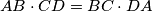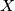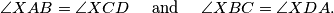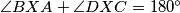### IMO Shortlist 2018 problem G6

Kvaliteta:
Avg: 0,0
Težina:
Avg: 8,0

A convex quadrilateral$ABCD$ satisfies$AB\cdot CD = BC\cdot DA$. Point$X$ lies inside$ABCD$ so thatProve that$\angle{BXA} + \angle{DXC} = 180^\circ$.

Izvor: https://www.imo-official.org/problems/IMO2018SL.pdf